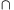Mathematical and Physical Journal
for High Schools
Issued by the MATFUND Foundation
 Already signed up? New to KöMaL?

#Problem A. 396. (March 2006)

A. 396. The elements of familiesandare finite sets, each having at most n elements. Suppose that for an arbitrary finite set F there existandsuch that XYF=Ø. Prove that there existandsuch that XY=Ø.

(5 pont)

Deadline expired on April 18, 2006.

### Statistics:

 6 students sent a solution. 5 points: Erdélyi Márton, Gyenizse Gergő, Nagy 224 Csaba, Paulin Roland. 3 points: 1 student. 2 points: 1 student.

Problems in Mathematics of KöMaL, March 2006